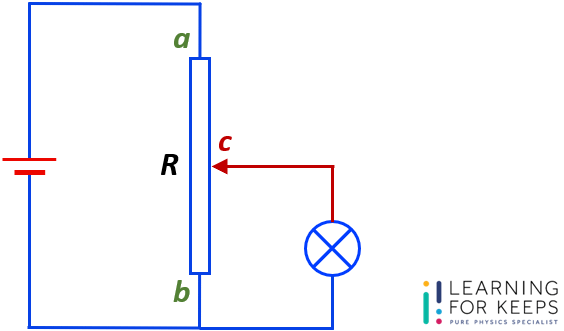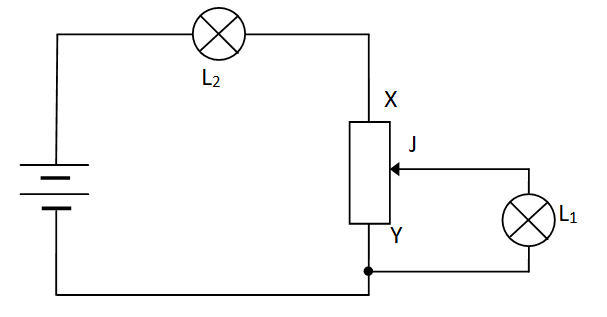# Quick Check Your Understanding of Physics Concepts

FEATURED

## Basic Physics Question Guide

Go through some of these questions and check your understanding of physics concepts

#### Topics: DC CircuIt – Resistance of potential divider circuit

The figure shows a circuit that uses a potential divider R.Assume that the density of wood is approximately 1.01 g/cm3.
What is the density of rubber?The resistance of R is 10 Ω.
R is divided into 2 sections, ac and bc, by the slider c.
Section ac of R has a resistance of 3.2 Ω.
The resistance of the lamp is also 3.2 Ω.
Calculate the total resistance of the circuit.

#### Topics: DC CircuIt

The diagram shows two identical lamps L1 and L2 connected to a rheostat and a battery.What happens to the brightness of the lamps as the contact J is moved towards the end X of the rheostat?

L1 L2
A brighter dimmer
B brighter brighter
C dimmer brighter
D dimmer dimmer

#### Topics: PRESSURE

A fork tube contains air in one branch and a vacuum in the other as shown below.What is the pressure of the enclosed air?

A d cm Hg
B k cm Hg
C (hd) cm Hg
D (hk)cm Hg

#### Topics: Forces Action-Reaction Pairs

The figure below shows a person sitting on a box that rests on the ground.Which of the following action-reaction pairs is correct?

A The weight of the man and the normal force from the box supporting man.
B The weight of the man and the contact force on the box by the man.
C The weight of the box and the normal force from the ground on the box.
D The weight of the man and the gravitational force of man on Earth.

#### Topics: TURNING EFFECT OF FORCES

The figure shows a light rod with two weights hanging from it. The rod is pivoted at its centre and the system is not in equilibrium. What is the moment required to bring the system into equilibrium?Moment Direction
A 2Nm clockwise
B 2Nm anticlockwise
C 4Nm clockwise
D 6Nm

anticlockwise

## We wish you all the best!

Quick check on Basic Physics Concept ultima modifica: 2019-10-20T02:25:55+00:00 da